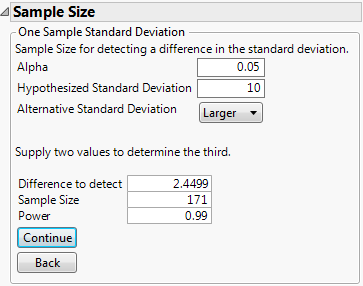Publication date: 04/12/2021

## Example of the One Sample Standard Deviation Calculator

Note: This example is from the online manual of The National Institute of Standards and Technology (NIST). You can access the NIST manual examples at https://www.itl.nist.gov/div898/handbook/prc/section2/prc232.htm.

The variance for resistivity measurements on a lot of silicon wafers is claimed to be 100 ohm-cm squared. The buyer is unwilling to accept a lot if the variance is greater than 155 ohm-cm squared. How many wafers must you test to estimate the lot variance with precision to detect an increase in 55 ohm-cm squared from the target of 100 ohm-cm squared? In terms of standard deviation, the hypothesized standard deviation, σ0, is 10 (the square root of 100) and σ is 12.4499 (the square root of 100 + 55 = 155). The difference to detect is 12.4499 – 10 = 2.4499. The desired power is 0.99 and the significance level is 0.05.

1. Select DOE > Design Diagnostics > Sample Size and Power.

2. Click One Sample Standard Deviation.

3. Leave Alpha as 0.05.

4. Enter 10 for Hypothesized Standard Deviation.

5. Select Larger for Alternative Standard Deviation.

6. Enter 2.4499 for Difference to Detect.

7. Leave Sample Size blank.

8. Enter 0.99 for Power.

9. Click Continue.

Figure 17.11 One-Sample Standard Deviation CalculatorYou must test 171 wafers in order to have a 99% chance of detecting an increase in the standard deviation of 2.4499 from a standard deviation of 10, with an alpha of 0.05.

Want more information? Have questions? Get answers in the JMP User Community (community.jmp.com).